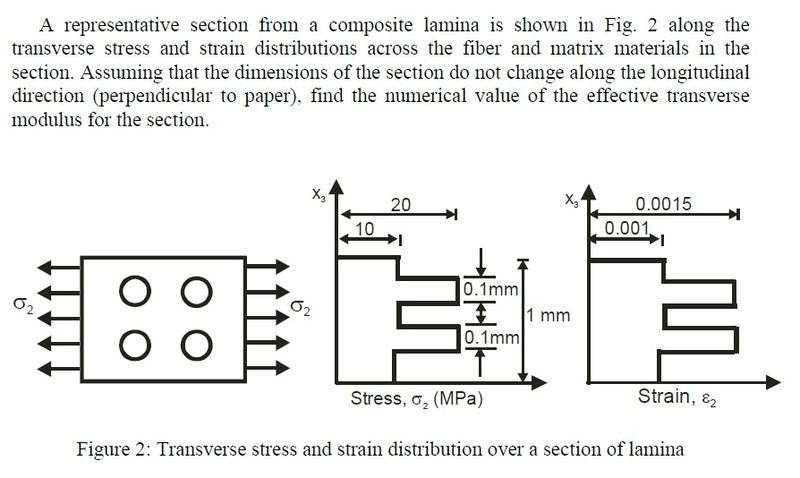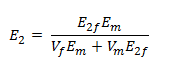# Find Numerical Value Of Transverse Modulus

newhere

## Homework Statement## Homework EquationsSquare Packing: V_f = ∏/4(r/R)^2
E=σ/ε

## The Attempt at a Solution

E=10MPa/0.001 = 1x10^4
E_2f=?
V_m=?

I get stuck here, am I on the right track with this problem? I need some guidance, please!